# 2NO(g)02(g)2NO2(g) AH° =-114.2 kJ and AS° =-146.5 J/K The equilibrium constant for this reaction at 321.0...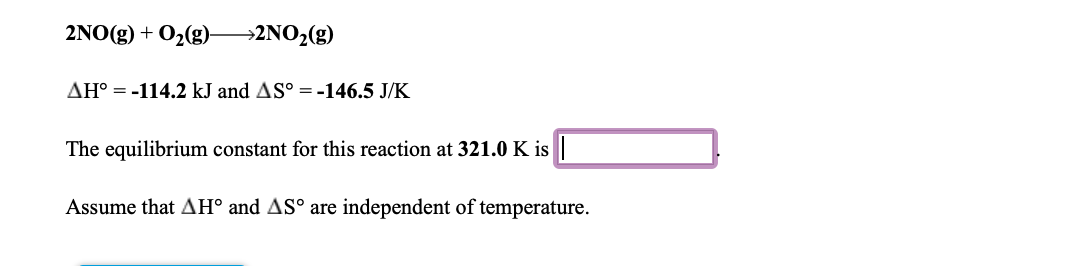2NO(g)02(g)2NO2(g) AH° =-114.2 kJ and AS° =-146.5 J/K The equilibrium constant for this reaction at 321.0 K is Assume that AH° and AS° are independent of temperature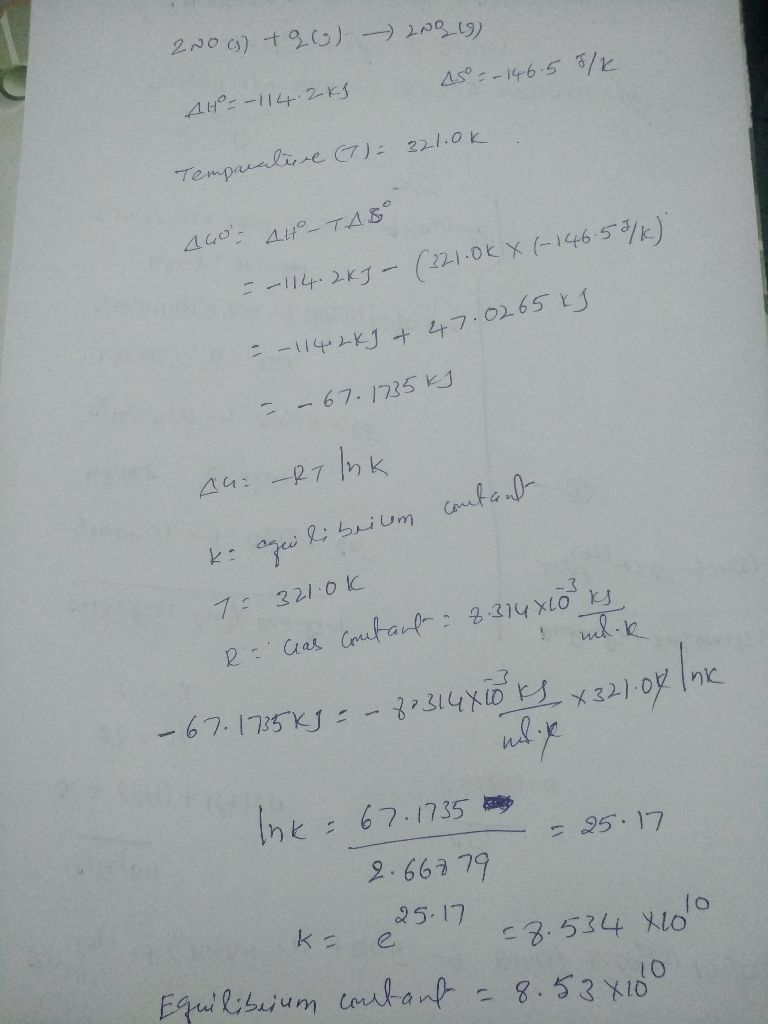#### Earn Coin

Coins can be redeemed for fabulous gifts.

Similar Homework Help Questions
• ### For the reaction 2NO(g) + O2(g) —>2NO2(g) AH° = -114.2 kJ and AS° = -146.5 J/K...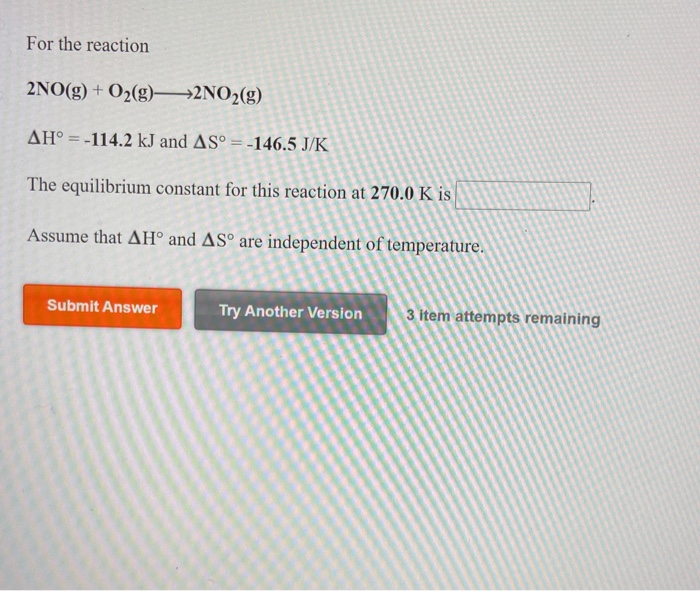For the reaction 2NO(g) + O2(g) —>2NO2(g) AH° = -114.2 kJ and AS° = -146.5 J/K The equilibrium constant for this reaction at 270.0 K is Assume that AH° and AS are independent of temperature. Submit Answer Try Another Version 3 item attempts remaining elefchces to access important values if needed for this question, Consider the reaction 2CO2(g) + 5H2(g)CH2(g) + 4H2O(g) Using the standard thermodynamic data in the tables linked above, calculate AG for this reaction at 298.15K if...

• ### For the reaction N2(g) + O2(g)->2NO(g) AH° = 180.6 kJ and AS = 24.9 J/K The...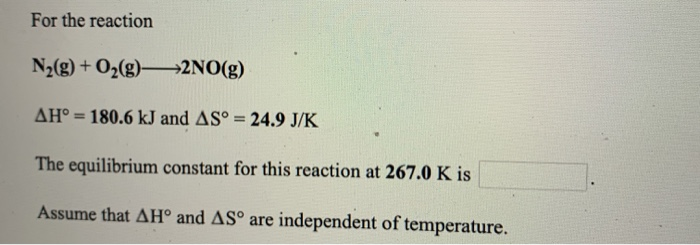For the reaction N2(g) + O2(g)->2NO(g) AH° = 180.6 kJ and AS = 24.9 J/K The equilibrium constant for this reaction at 267.0 K is Assume that AHⓇ and ASº are independent of temperature.

• ### For the reaction N2(g) + O2(g)2NO(g) AH° - 180.6 kJ and AS - 24.9 J/K The...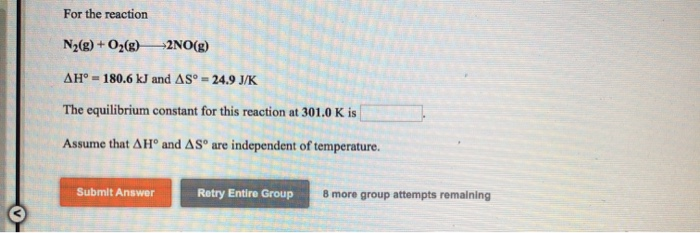For the reaction N2(g) + O2(g)2NO(g) AH° - 180.6 kJ and AS - 24.9 J/K The equilibrium constant for this reaction at 301.0K is Assume that A Hº and AS are independent of temperature. Submit Answer Retry Entire Group 8 more group attempts remaining

• ### pt Use the References to access important values if needed for pt For the reaction pt...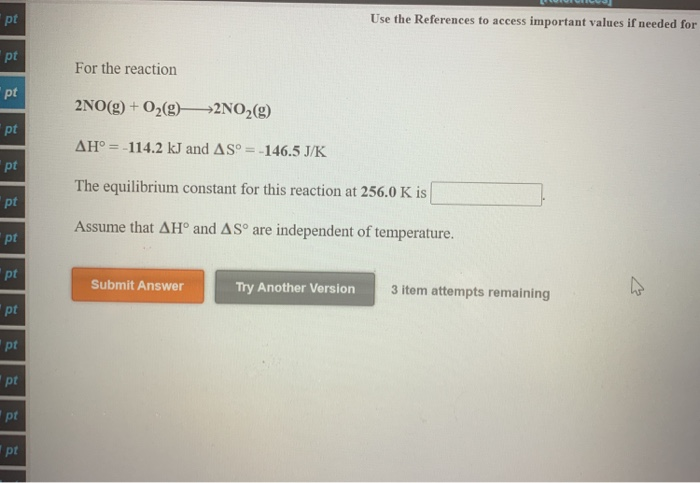pt Use the References to access important values if needed for pt For the reaction pt 2NO(g) + O2(g)—>2NO2(g) pt AH° = -114.2 kJ and AS = -146.5 J/K pt The equilibrium constant for this reaction at 256.0 K is pt Assume that AHⓇ and AS are independent of temperature. pt pt Submit Answer Try Another Version 3 item attempts remaining pt pt pt - pt pr

• ### 3. Consider the chemical equilibrium for the following chemical reaction AH -+114 kJ 2NO2(g) 2NO(g) +...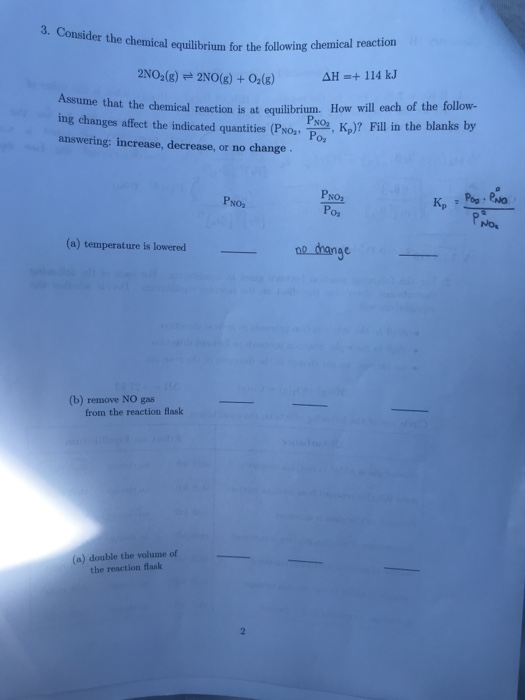3. Consider the chemical equilibrium for the following chemical reaction AH -+114 kJ 2NO2(g) 2NO(g) + 02(s) Assume that the chemical reaction is at equilibrium. How will each of the follow- Kp)? Fill in the blanks by PNO ing changes affect the indicated quantities (PNo2 Po answering: increase, decrease, or no change. Poa PO К, PNO2 Pos PNO2 PM No hange (a) temperature is lowered (b) remove NO gas from the reaction flask (a) double the volume of the reaction...

• ### (a) For the reaction 2NO2 (g) 2NO (g) + 02 (g) K # 0.50. Predict the...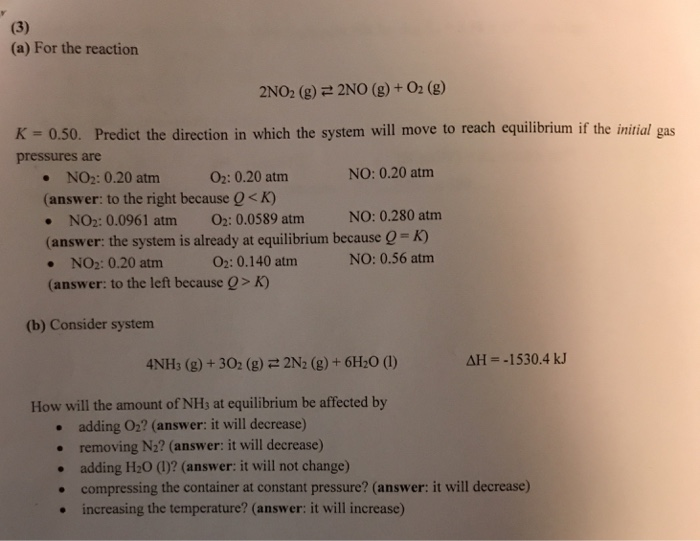(a) For the reaction 2NO2 (g) 2NO (g) + 02 (g) K # 0.50. Predict the direction in which the system will move to reach equilibrium if the initial gas pressures are NO: 0.20 atm . NO2: 0.20 atm (answer: to the right because Q< K) .NO2: 0.0961 atm 02: 0.0589 atm NO: 0.280 atm (answer: the system is already at equilibrium because Q -K) O2: 0.20 atm NO: 0.56 atm NO2: 0.20 atm (answer: to the left because Q>...

• ### Given the following reactions Question 1 Given the following reactions AH 66.4 k N2 (g)+202 (g)2NO2...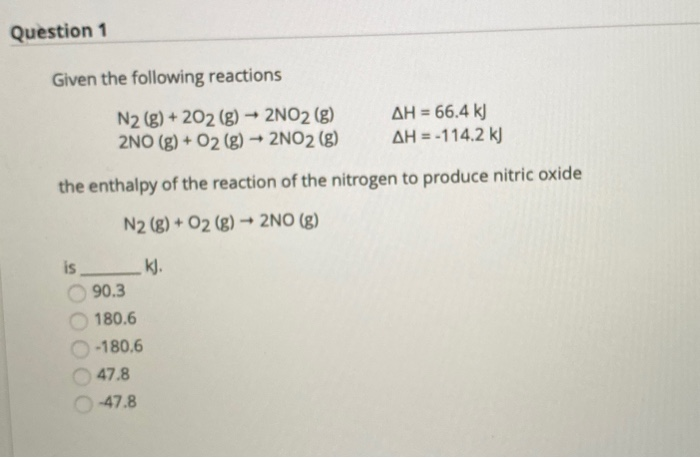Given the following reactions Question 1 Given the following reactions AH 66.4 k N2 (g)+202 (g)2NO2 (g) 2NO (g)+02 (g) 2NO2 (g) AH=-114.2 k the enthalpy of the reaction of the nitrogen to produce nitric oxide 2NO (g) N2 (g)+02 (g) KJ. is 90.3 180.6 -180.6 47.8 47.8

• ### For the reaction 2802(g) + O2(g)—>2503(g) AH° = -197.8 kJ and AS° = -187.9 J/K The...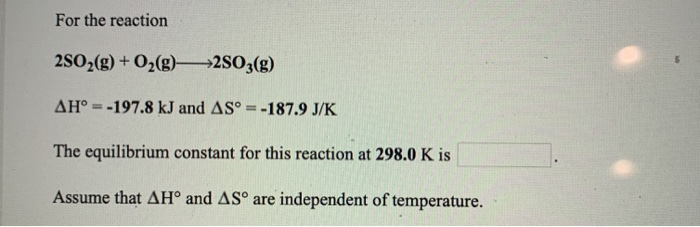For the reaction 2802(g) + O2(g)—>2503(g) AH° = -197.8 kJ and AS° = -187.9 J/K The equilibrium constant for this reaction at 298.0 K is Assume that AHⓇ and AS™ are independent of temperature.

• ### For the reaction 2NH3(g) 202(g)N20(g)+3H20(I) AHo 683 kJ and AS° = -366 J/K Kelvin The equilibrium constant, K, would b...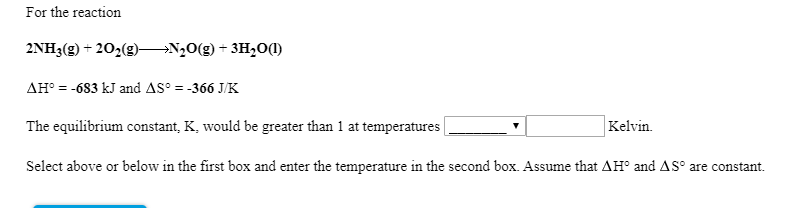For the reaction 2NH3(g) 202(g)N20(g)+3H20(I) AHo 683 kJ and AS° = -366 J/K Kelvin The equilibrium constant, K, would be greater than 1 at temperatures Select above or below in the first box and enter the temperature in the second box. Assume that AH° and AS° are constant For the reaction N2(g)O2(g)>2NO(g) AH 181 kJ and AS° = 24.9 J/K The equilibrium constant, K, would be greater than 1 at temperatures Kelvin Select above or below in the first box...

• ### 4. For the reaction Ny() + O2()—>2NO(g) AH-181 kJ and AS - 24.9 J/K AGⓇ would...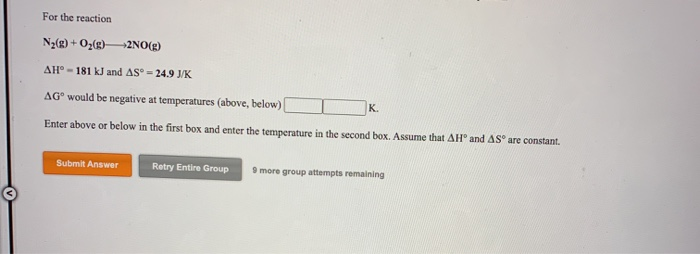4. For the reaction Ny() + O2()—>2NO(g) AH-181 kJ and AS - 24.9 J/K AGⓇ would be negative at temperatures (above, below) Enter above or below in the first box and enter the temperature in the second box. Assume that AHⓇ and AS are constant. K Submit Answer Retry Entire Group 9 more group attempts remaining For the reaction CaCO3(s) CaO(s) + CO2(g) AH° -178 kJ and AS - 161 J/K AGⓇ would be negative at temperatures (above, below) K...

Free Homework App